## 使用优化实时编辑器任务或求解器的有约束非线性问题

### 问题表示：罗森布罗克函数

`$f\left(x\right)=100{\left({x}_{2}-{x}_{1}^{2}\right)}^{2}+{\left(1-{x}_{1}\right)}^{2},$`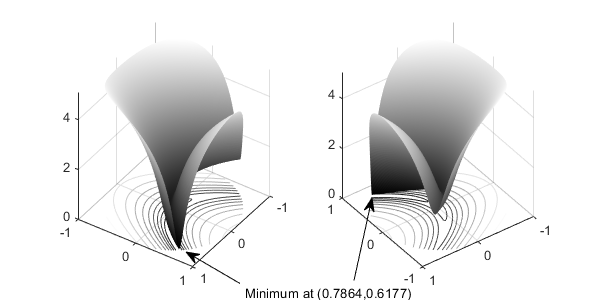### 使用优化实时编辑器任务定义和求解问题

1. 点击主页选项卡文件部分的新建实时脚本按钮，创建一个新的实时脚本。2. 插入一个优化实时编辑器任务。点击插入选项卡，然后在代码部分中选择任务 > 优化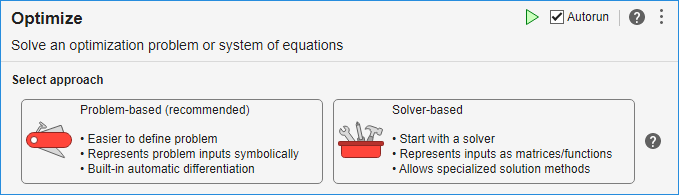3. 选择基于求解器的任务。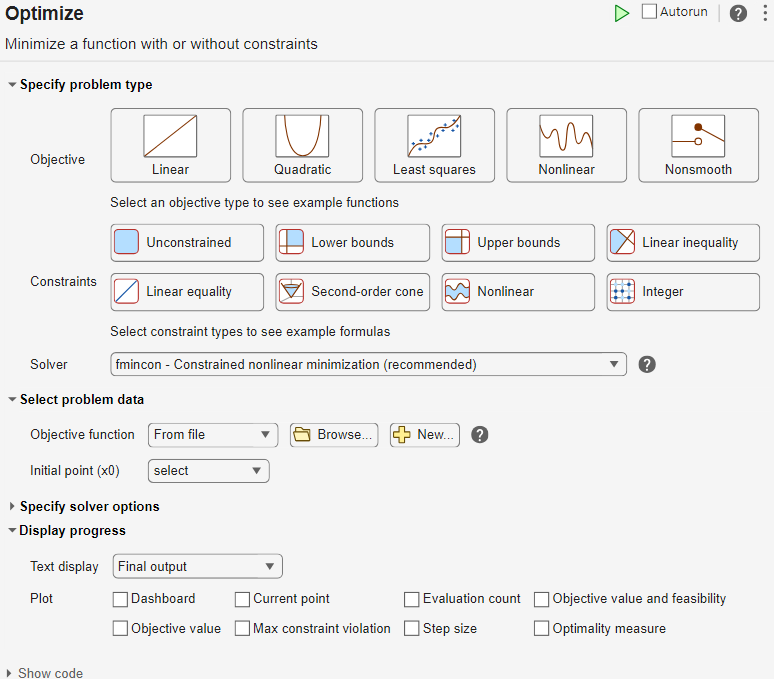4. 在任务的指定问题类型节中，选择目标 > 非线性约束 > 非线性。该任务选择求解器 fmincon - 有约束非线性最小化

5. 包括罗森布罗克函数作为目标函数。在任务的选择问题数据节中，选择目标函数 > 局部函数，然后点击 按钮。新局部函数出现在任务下的一个节中。

```function f = objectiveFcn(optimInput) % Example: % Minimize Rosenbrock's function % f = 100*(y - x^2)^2 + (1 - x)^2 % Edit the lines below with your calculation x = optimInput(1); y = optimInput(2); f = 100*(y - x^2)^2 + (1 - x)^2; end```

此函数实现罗森布罗克函数。

6. 在任务的选择问题数据节中，选择目标函数 > objectiveFcn

7. 将初始点 `x0 = [0;0]` 放入 MATLAB 工作区中。通过点击任务，然后点击插入选项卡上的按钮，在优化任务上方插入一个新节。在任务上方的新节中，输入以下初始点代码。

`x0 = [0;0];`
8. 通过按 Ctrl+Enter 运行该节。此操作会将 `x0` 放入工作区中。

9. 在任务的选择问题数据节中，选择初始点(x0) > x010. 选择问题数据节中，选择约束 > 非线性 > 局部函数，然后点击 按钮。新局部函数出现在前一个局部函数的下方。

11. 按如下所示编辑新局部函数。

```function [c,ceq] = unitdisk(x) c = x(1)^2 + x(2)^2 - 1; ceq = [ ]; end```
12. 选择问题数据节中，选择 unitdisk 作为约束函数。13. 要监控求解器进度，请在任务的显示进度节中，选择文本显示 > 每次迭代。此外，为绘图选择14. 要运行求解器，请点击任务窗口右上角的选项按钮 ，并选择运行节。绘图出现在单独的图窗窗口中和输出区域中。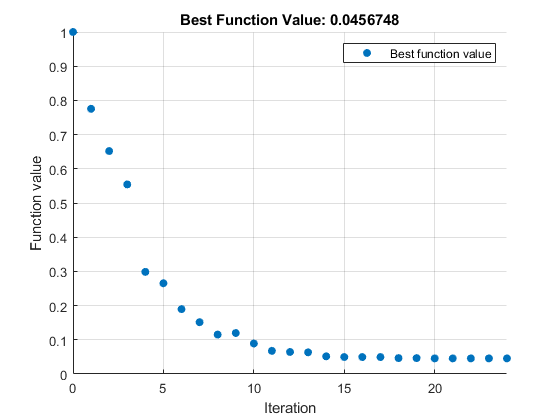输出区域显示一个迭代表，解释结果中有对它的进一步说明。

15. 要找到解，请查看任务的顶部。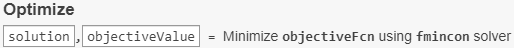求解器将变量 `solution``objectiveValue` 放置在工作区中。通过在任务下方插入新分节符并输入以下行来查看其值。16. 通过按 Ctrl+Enter 运行该节。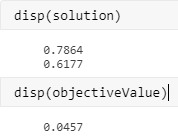要了解 `fmincon` 得到结果的过程，请参阅解释结果

17. 要显示优化为求解问题而生成的代码，请点击任务窗口右上角的选项按钮 ，并选择控件和代码在任务的底部，出现以下代码。

```% Set nondefault solver options options = optimoptions('fmincon','Display','iter','PlotFcn',... 'optimplotfvalconstr'); % Solve [solution,objectiveValue] = fmincon(@objectiveFcn,x0,[],[],[],[],[],[],... @unitdisk,options);```

此代码是您在命令行中用于求解问题的代码，如下节中所述。

### 在命令行中定义和求解问题

`[x,fval] = fmincon(fun,x0,A,b,Aeq,beq,lb,ub,nonlcon,options)`

`fun``nonlcon` 输入分别表示目标函数和非线性约束函数。

1. 以 MATLAB 语言将目标函数定义为函数文件或匿名函数。此示例使用一个函数文件。

2. 将约束定义为一个单独的文件或匿名函数。

1. 在命令行中输入：

`edit rosenbrock`
2. 在 MATLAB 编辑器中输入：

```%% ROSENBROCK(x) expects a two-column matrix and returns a column vector % The output is the Rosenbrock function, which has a minimum at % (1,1) of value 0, and is strictly positive everywhere else. function f = rosenbrock(x) f = 100*(x(:,2) - x(:,1).^2).^2 + (1 - x(:,1)).^2;```

注意

`rosenbrock` 是向量化函数，它可以一次计算多个点处的值。请参阅向量化。向量化函数最适合绘图。对于非向量化版本，请输入：

```%% ROSENBROCK1(x) expects a two-element vector and returns a scalar % The output is the Rosenbrock function, which has a minimum at % (1,1) of value 0, and is strictly positive everywhere else. function f = rosenbrock1(x) f = 100*(x(2) - x(1)^2)^2 + (1 - x(1))^2;```
3. 用名称 `rosenbrock.m` 保存文件。

1. 创建一个名为 `unitdisk.m` 的文件，其中包含以下代码：

```function [c,ceq] = unitdisk(x) c = x(1)^2 + x(2)^2 - 1; ceq = [ ];```
2. 保存 `unitdisk.m` 文件。

3. 创建目标函数和约束函数的函数句柄。这些函数句柄指向函数，是求解器访问函数的一种方式。

```fun = @rosenbrock; nonlcon = @unitdisk;```

1. `fmincon` 创建选项，以使用 `'optimplotfvalconstr'` 绘图函数并返回迭代输出。

```options = optimoptions('fmincon',... 'PlotFcn','optimplotfvalconstr',... 'Display','iter');```
2. 创建初始点。

`x0 = [0 0];`
3. 为此示例不使用的约束创建空项。

```A = []; b = []; Aeq = []; beq = []; lb = []; ub = [];```

`[x,fval] = fmincon(fun,x0,A,b,Aeq,beq,lb,ub,nonlcon,options)`
``` First-order Norm of Iter F-count f(x) Feasibility optimality step 0 3 1.000000e+00 0.000e+00 2.000e+00 1 13 7.753537e-01 0.000e+00 6.250e+00 1.768e-01 2 18 6.519648e-01 0.000e+00 9.048e+00 1.679e-01 3 21 5.543209e-01 0.000e+00 8.033e+00 1.203e-01 4 24 2.985207e-01 0.000e+00 1.790e+00 9.328e-02 5 27 2.653799e-01 0.000e+00 2.788e+00 5.723e-02 6 30 1.897216e-01 0.000e+00 2.311e+00 1.147e-01 7 33 1.513701e-01 0.000e+00 9.706e-01 5.764e-02 8 36 1.153330e-01 0.000e+00 1.127e+00 8.169e-02 9 39 1.198058e-01 0.000e+00 1.000e-01 1.522e-02 10 42 8.910052e-02 0.000e+00 8.378e-01 8.301e-02 11 45 6.771960e-02 0.000e+00 1.365e+00 7.149e-02 12 48 6.437664e-02 0.000e+00 1.146e-01 5.701e-03 13 51 6.329037e-02 0.000e+00 1.883e-02 3.774e-03 14 54 5.161934e-02 0.000e+00 3.016e-01 4.464e-02 15 57 4.964194e-02 0.000e+00 7.913e-02 7.894e-03 16 60 4.955404e-02 0.000e+00 5.462e-03 4.185e-04 17 63 4.954839e-02 0.000e+00 3.993e-03 2.208e-05 18 66 4.658289e-02 0.000e+00 1.318e-02 1.255e-02 19 69 4.647011e-02 0.000e+00 8.006e-04 4.940e-04 20 72 4.569141e-02 0.000e+00 3.136e-03 3.379e-03 21 75 4.568281e-02 0.000e+00 6.440e-05 3.974e-05 22 78 4.568281e-02 0.000e+00 8.000e-06 1.084e-07 23 81 4.567641e-02 0.000e+00 1.601e-06 2.793e-05 24 84 4.567482e-02 0.000e+00 2.023e-08 6.916e-06 Local minimum found that satisfies the constraints. Optimization completed because the objective function is non-decreasing in feasible directions, to within the value of the optimality tolerance, and constraints are satisfied to within the value of the constraint tolerance. x = 0.7864 0.6177 fval = 0.0457```### 解释结果

• 第一列（标记为 `Iter`）是从 0 到 24 的迭代序号。`fmincon` 经过 24 次迭代后收敛。

• 第二列（标记为 `F-count`）报告计算罗森布罗克函数的累计次数。最后一行显示 `F-count` 为 84，表明 `fmincon` 在求解最小值的过程中对罗森布罗克函数进行了 84 次计算。

• 第三列（标记为 `f(x)`）显示目标函数值。最终值 `4.567482e-2` 是在优化运行中报告的最小值，位于命令行窗口中退出消息的末尾。

• 第四列 `Feasibility` 对于所有迭代均为 0。此列显示在约束为正的每次迭代中约束函数 `unitdisk` 的值。由于 `unitdisk` 的值在所有迭代中均为负数，因此每次迭代都满足约束。